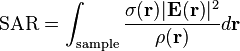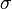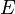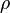## Friday, December 19, 2014

### Check SAR Value

Specific absorption rate (SAR) is a measure of the rate at which energy is absorbed by the human body when exposed to a radio frequency (RF) electromagnetic field; although, it can also refer to absorption of other forms of energy by tissue, including ultrasound. It is defined as the power absorbed per mass of tissue and has units of watts per kilogram (W/kg). SAR is usually averaged either over the whole body, or over a small sample volume (typically 1 g or 10 g of tissue). The value cited is then the maximum level measured in the body part studied over the stated volume or mass.

SAR for electromagnetic energy can be calculated from the electric field within the tissue as:$\text{SAR} = \int_\textrm{sample} \frac{\sigma(\mathbf{r}) |\mathbf{E}(\mathbf{r})|^2}{\rho(\mathbf{r})} d\mathbf{r}$
where$\sigma$ is the sample electrical conductivity$E$ is the RMS electric field$\rho$ is the sample density
You can check Radiation level in terms of SAR of your smartphone by dialing a USSD code *#07#, if results shows SAR below 1.6 watts per kilogram (1.6 W/kg) then it is OK otherwise you are advised to change your smartphone immediately.

Related Article:- http://www.knowcrazy.com/2011/04/check-your-own-mobile-number-msisdn.html